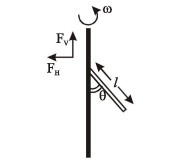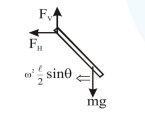# Prove the following

Question:A uniform rod of length ' $l$ ' is pivoted at one of its ends on a vertical shaft of negligible radius. When the shaft rotates at angular speed $\omega$ the rod makes an angle $\theta$ with it (see figure). To find $\theta$ equate the rate of change of angular momentum (direction going into the paper)

$\frac{m \ell^{2}}{12} \omega^{2} \sin \theta \cos \theta$ about the centre of mass

(CM) to the torque provided by the horizontal and vertical forces $\mathrm{F}_{\mathrm{H}}$ and $\mathrm{F}_{\mathrm{V}}$ about the $\mathrm{CM}$. The value of $\theta$ is then such that:

1. $\cos \theta=\frac{g}{2 \ell \omega^{2}}$

2. $\cos \theta=\frac{3 g}{2 \ell \omega^{2}}$

3. $\cos \theta=\frac{2 \mathrm{~g}}{3 \ell \omega^{2}}$

4. $\cos \theta=\frac{\mathrm{g}}{\ell \omega^{2}}$

Correct Option: , 2

Solution:$\mathrm{F}_{\mathrm{V}}=\mathrm{mg}$

$\mathrm{F}_{\mathrm{H}}=\mathrm{m} \omega^{2} \frac{\ell}{2} \sin \theta$

$\mathrm{mg} \frac{\ell}{2} \sin \theta-\mathrm{m} \omega^{2} \frac{\ell}{2} \sin \theta \frac{\ell}{2} \cos \theta=\frac{\mathrm{m} \ell^{2}}{12} \omega^{2} \sin \theta \cos \theta$

$\cos \theta=\frac{3}{2} \frac{g}{\omega^{2} \ell}$ ..........(ii)